CS 112 Lab 8: Queues

Objectives:

In this exercise, you will:

1. Complete a dynamic-array-based Queue class.
2. Complete a linked-node-based Queue class.
3. Practice using user-defined exceptions.

Introduction

Today's exercise is to complete two dynamic queue classes:

1. an ArrayQueue class that stores items in a dynamic array; and
The exercise will provide you with test-classes and partially finished queue classes; your task is to complete the queue classes.

Getting Started

Begin by creating a new project for this week's files. Download tester.cpp, ArrayQueueTester.h, ArrayQueueTester.cpp, ArrayQueue.h, ArrayQueue.cpp, LinkedQueueTester.h, LinkedQueueTester.cpp, LinkedQueue.h, LinkedQueue.cpp, Exception.h, and QueueException.h. Import these files into your project, open the files, and personalize their opening documentation. Then take a few minutes to look them over, to become familiar with their contents.

The two exception files define a simple exception hierarchy, that we can visualize as shown in Figure 1:Figure 1. An Exception Hierarchy

This hierarchy provides us with finer-grained exception-handling: a method that sends the append() message can catch the FullQueueException, and a method that sends the remove() or getFirst() message can catch the EmptyQueueException. Be sure to look these over, as different constructors require different numbers of arguments.

Part I: The ArrayQueue Class

As you can see, our ArrayQueue class contains five instance variables:

```   unsigned myCapacity;       // how many items I can store
unsigned mySize;           // number of items I contain
unsigned myFirstIndex;     // index of oldest item (if any)
unsigned myLastIndex;      // index of next available spot for append (if any)
Item*    myArrayPtr;       // dynamic array of items
```
We will store the address of a dynamically allocated array in myArrayPtr, and we will store the length of that array in myCapacity. We will store the number of items in that array in mySize, store the index of the oldest item in that array in myFirstIndex, and store the index of the newest item in the array in myLastIndex.

Note that this is quite different from our implementation of class Stack last week. For the Stack, there was a direct relationship between the Stack's size attribute and the most recently added item (i.e., in a non-empty Stack, the expression mySize-1 was always the index of the top item). That relationship held for an array-based Stack because we always added and removed items from the same end of its array.

We need all of these instance variables to implement the Queue operations efficiently because we will add items to one end of the Queue's array (i.e., the tail of the queue) and remove items from the opposite end of its array (i.e., the head of the queue). That implies our Queue class needs to "remember" two index values: (i) the index of the oldest item in the queue (which we will store in myFirstIndex), and (ii) the index of the newest item in the queue (which we will store in myLastIndex).

To illustrate, if we have added two items -- 11 and 22 -- to our queue, we might visualize its structure as shown in Figure 2: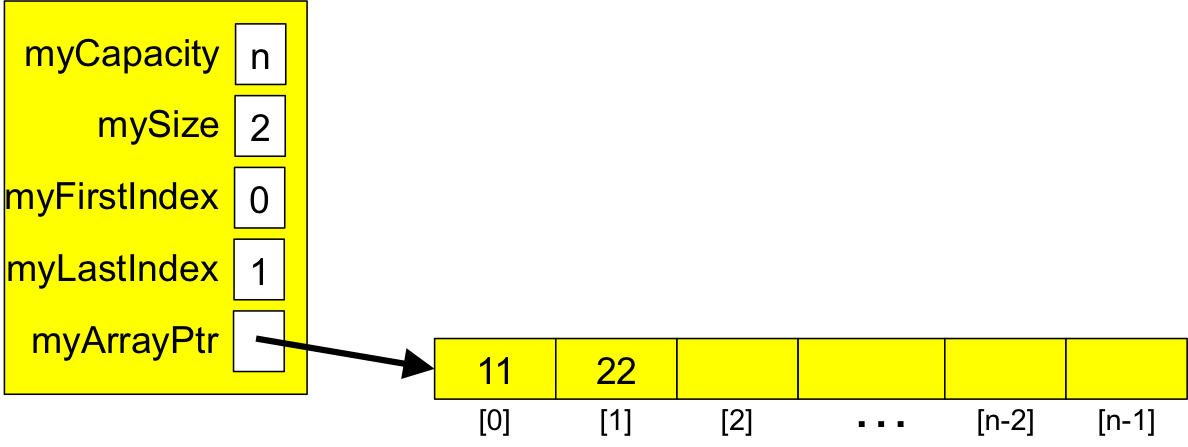Figure 2. An Array-based Queue Containing Two Items

The oldest item -- 11 -- is at index 0, and the newest item -- 22 -- is at index 1, so myFirstIndex is 0 and myLastIndex is 22.

The "trick" to using the array efficiently is to implement the queue operations using the modulus operator (%), so that these operations use the array in a circular fashion as shown in Figure 3: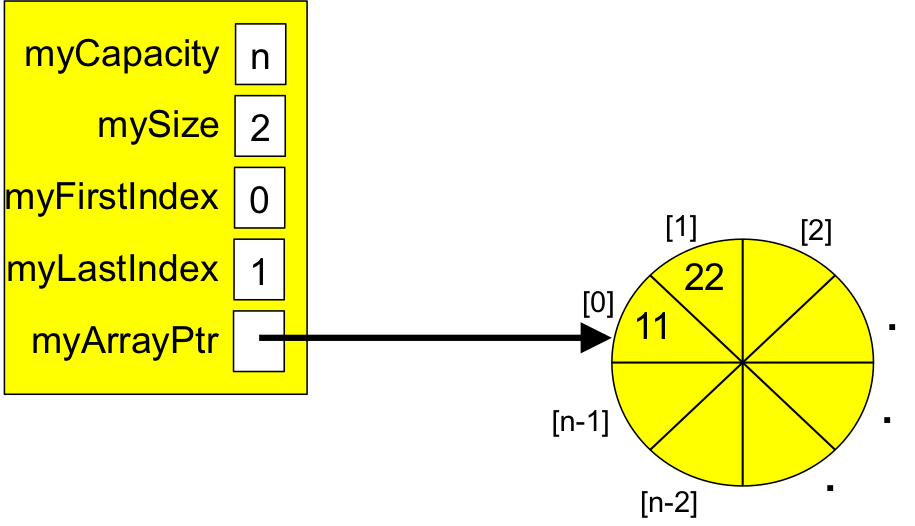Figure 3. An Array-based Queue As a Circular Queue

Then we just have to "advance" myLastIndex every time an item is added to the queue, and "advance" myFirstIndex every time an item is removed from the queue, all the while ensuring that mySize correctly reflects the number of items in the queue.

The primary queue operations are:

• bool isEmpty(), that returns true if and only if the queue contains no items;
• bool isFull(), that returns true if and only if the queue has no room for more items;
• void append(Item it), that places it on at the end of the queue;
• Item getFirst(), that returns the oldest item from the queue (without modifying the queue ); and
• Item remove(), that removes and returns the oldest item from the queue.

To narrow our focus, the provided queue classes already contain prototypes and definitions for:

• isEmpty() and isFull(), as described above;
• getSize(), that returns the number of items in the queue;
• a copy constructor;
• a destructor; and
• an assignment operator.
Run the project, and verify that everything works correctly at the outset.

The ArrayQueue Constructor

Uncomment the definition and call of ArrayQueueTester::testConstructor(). Take a few minutes to look over its definition, to see the tests it contains, specifically how it constructs a queue. Our ArrayQueue constructor must initialize the members of an ArrayQueue with values appropriate for an initially empty queue.

Compile your project. You should see an error indicating that there is no constructor matching the calls in our test-method.

In ArrayQueue.h, add a prototype for this constructor to the class. Recompile and rerun. If you have done it correctly, you should now see a linking error, since our constructor has been prototyped but not defined.

To define the constructor, it may not be obvious what the values of some of the instance variables should be. For example, suppose we define an ArrayQueue as follows:

```    ArrayQueue aq(4);
```
Our argument 4 tells the constructor that we want a queue whose capacity is 4, and since it is initially empty, its size should be 0, but what should the initial values of myFirstIndex and myLastIndex be? Figure 4 shows our situation: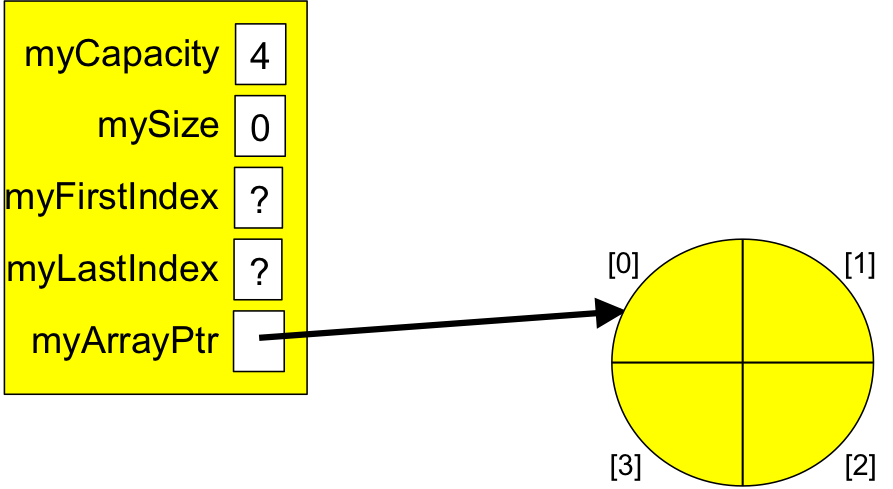Figure 4. Initializing an Array-based Queue of Capacity 4.

To answer this question, let's walk through our use of the queue. If we add the item 11 to the queue, our queue should appear as shown in Figure 5: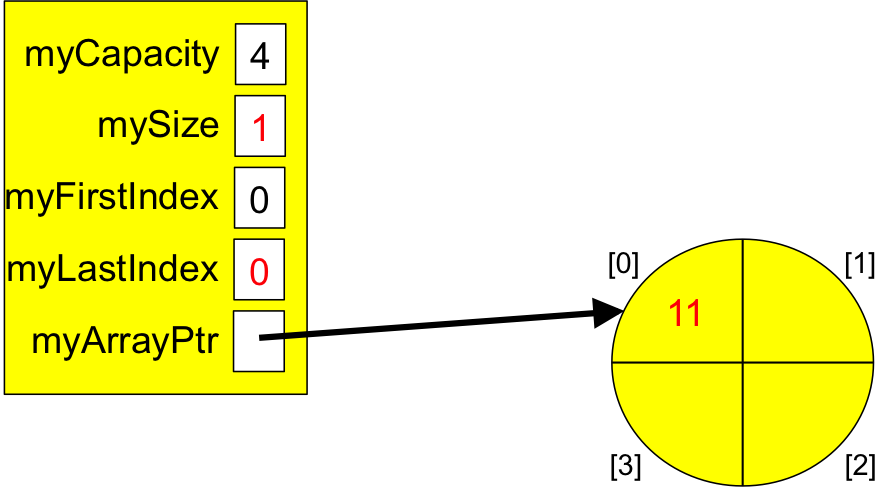Figure 5. Our Array-based Queue Containing 1 Item.

If we then add 22 to the queue, it should appear as shown in Figure 6:Figure 6. Our Array-based Queue Containing 2 Items.

If we then add 33 to the queue, it should appear as shown in Figure 7: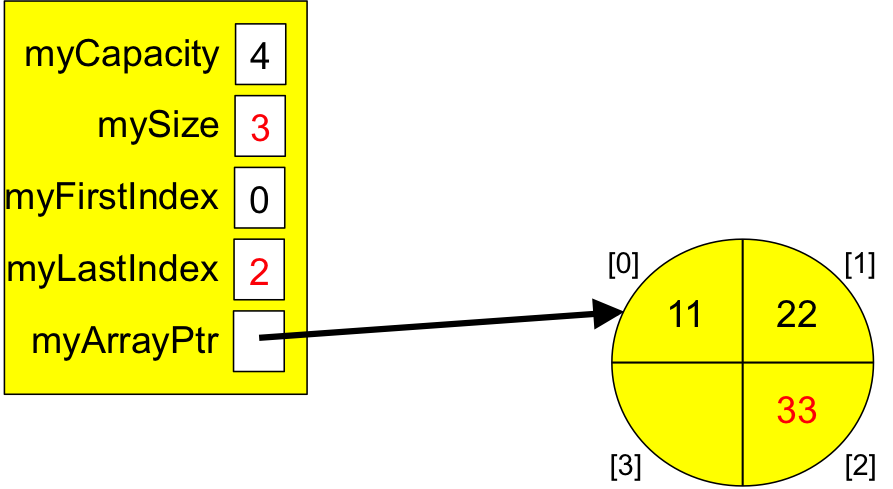Figure 7. Our Array-based Queue Containing 3 Items.

If we then add 44 to the queue, it should appear as shown in Figure 8:Figure 8. Our Array-based Queue Containing 4 Items.

Note that our queue is now full; any further attempts to add an item should throw the FullQueueException shown in Figure 1.

Now, let's start removing items and do so until the queue is empty. Removing the oldest item will return the 11, leaving our queue as shown in Figure 9: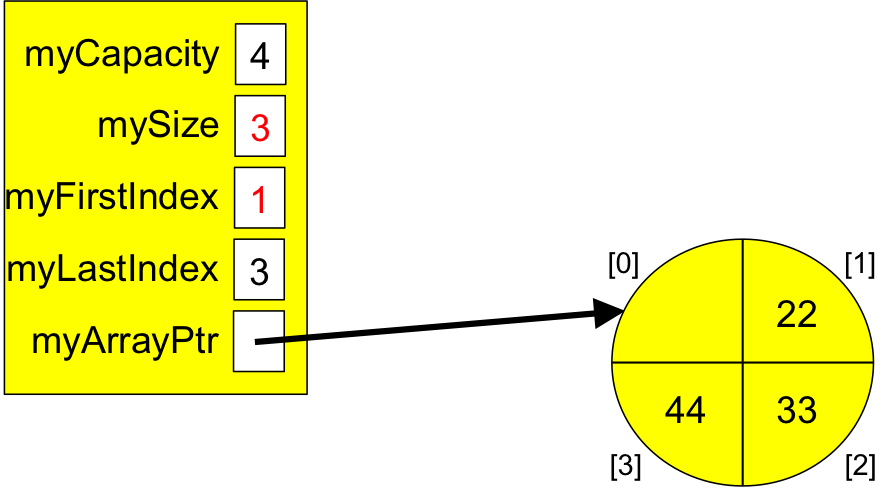Figure 9. Our Array-based Queue Containing 3 Items.

Removing the next-oldest item should return the 22, leaving our queue as shown in Figure 10:Figure 10. Our Array-based Queue Containing 2 Items.

Removing the next-oldest item should return the 33, leaving our queue as shown in Figure 11: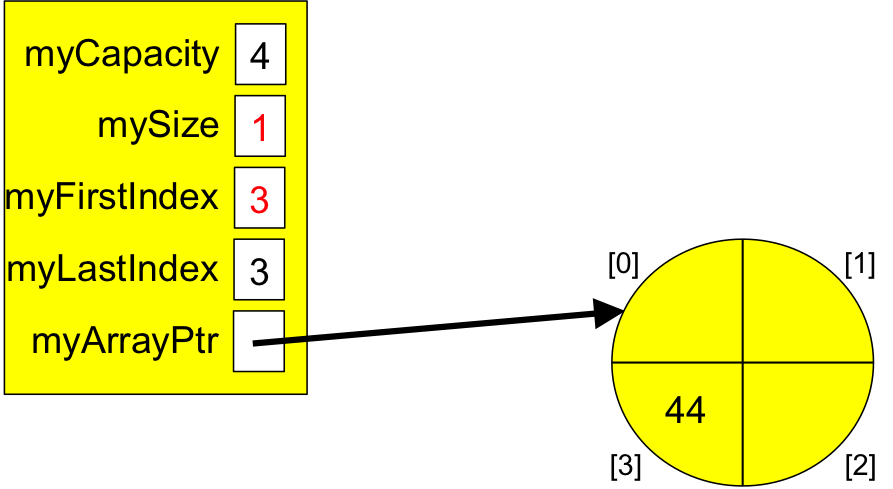Figure 11. Our Array-based Queue Containing 1 Item.

Removing the final item should return the 44, leaving our queue as shown in Figure 12: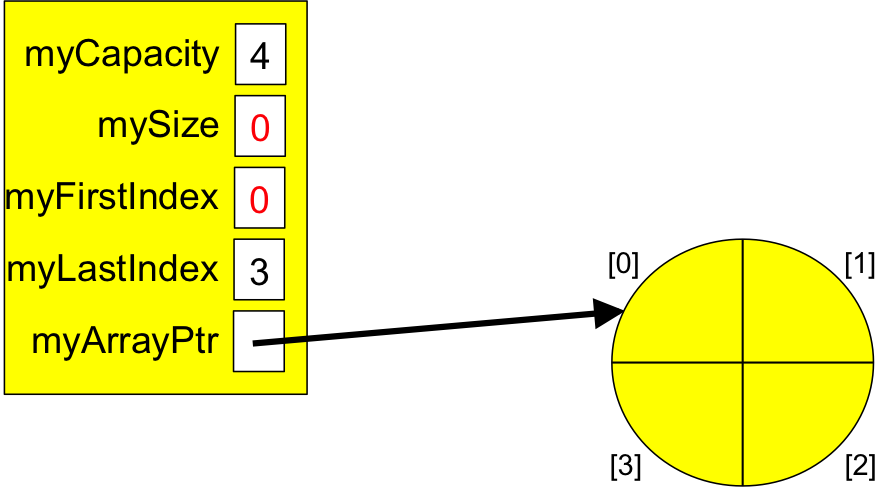Figure 12. Our Empty Array-based Queue.

Note that the queue shown in Figure 12 is empty. and it shows valid values for myFirstIndex and myLastIndex. We can thus use this information to initialize these two instance variables and answer the question posed in Figure 4.

In ArrayQueue.cpp, add a definition for this constructor that will pass testConstructor().

Note that your definition should initialize myLastIndex correctly relative to capacity. For example, if capacity is 8, you should not be initializing myLastIndex to 3...

If you get stuck, here is a hint, but don't use it unless you have to.

Recompile and rerun your test. Continue when you pass the test.

The append(), getFirst(), and getLast() Methods

Before we can test the other operations, we need to be able to add items to a queue, so we now turn our attention to the append() method. Since adding items to a queue makes it non-empty, we will also define the getFirst() and getLast() methods.

In ArrayQueueTester.cpp, uncomment the call to and definition of the testAppend() method. Compile and run the test. You should see error messages indicating that append(), getFirst(), and getLast() do not exist.

In ArrayQueue.h, add a prototype for each of these methods to the class. Recompile. You should now get linking errors, since these methods have been declared but not defined.

The getFirst() Method. In ArrayQueue.cpp, add a definition for getFirst(). This definition should be fairly simple, throwing an EmptyQueueException if the queue is empty; otherwise, it should return the item at index myFirstIndex in myArrayPtr.

Save/compile, and verify that the linking error for getFirst() goes away. (The errors for getLast() and append() should still be there.)

The getLast() Method. Add a definition for getLast(). This method should follow the same pattern as getFirst(): if the queue is empty, throw an EmptyQueueException; otherwise, return the item at index myLastIndex within myArrayPtr.

Save/compile, and verify that the linking error for getLast() goes away. (The error for append() should still be there.)

The append() Method

To figure out how the append() method should work, take a moment to review Figures 4 through 8, in which we filled our queue with items, and then Figures 9 and 10, in which we removed the two oldest items. Here is Figure 10 again:Figure 10. Our Array-based Queue Containing 2 Items.

Now, suppose that instead of removing the remaining two items, we add 55 to our queue? Since there is space available, we should "wrap around the circle" and place the 55 at index 0, as shown in Figure 13: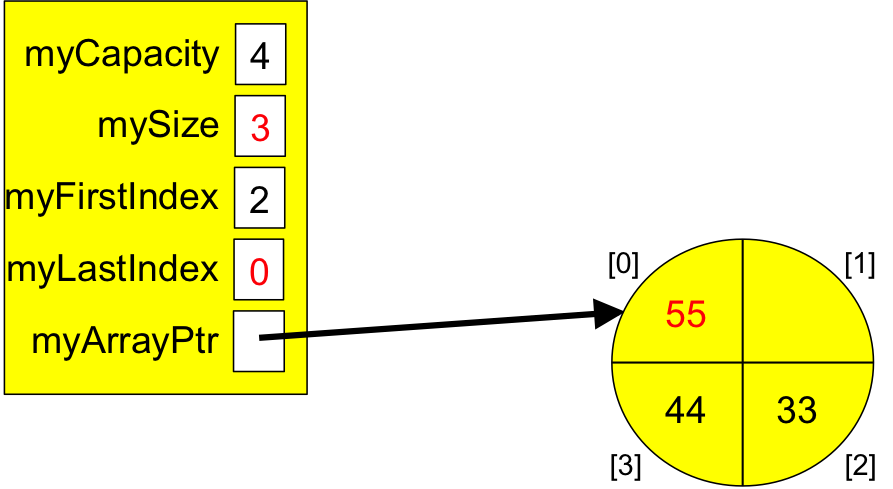Figure 13. Our Array-based Queue Containing 33, 44, and 55.

Again, suppose that we add 66 to our queue? Figure 14 shows how things should appear: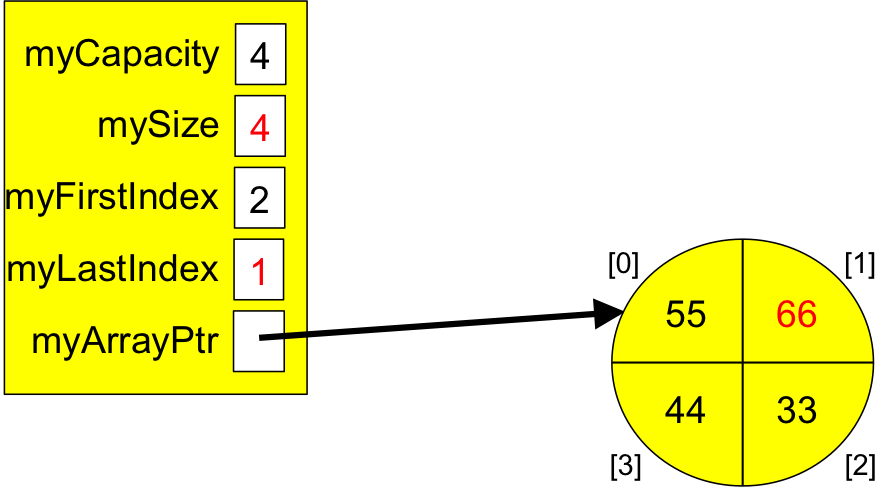Figure 14. Our Array-based Queue Containing 33, 44, 55, and 66.

Our queue is now full again, so we cannot add more items until some have been removed.

Some of the key things to observe are that the append() operation:

• only changes myLastIndex and mySize, not myFirstIndex;
• needs to make the myLastIndex member "wrap around" to 0 when it reaches myCapacity, which can be done using the modulus operator (%).

Using this information, define the append() method. Save/recompile, and verify that what you have written compiles without errors.

Run the tests. If you pass the tests, congratulations! If not, go back and fix your append(), getFirst() and/or getLast() methods. If you get stuck, here is a hint for append(), but only use it if you have to.

Continue when your methods pass all the tests.

The remove() Method

Now that we are able to add items to our ArrayQueue, and view the first and last items in the queue, all that remains is to build the remove() operation.

In ArrayQueueTester.cpp, uncomment the call to and definition of the testRemove() method. Compile and run the test. You should see an error message indicating that remove() does not exist.

In ArrayQueue.h, add a prototype for remove() to the class. Recompile and rerun. You should now see a linking error, since remove() has been prototyped but not defined.

To see how remove() should be behave, let's continue where we left off in Figure 14. Here it is again:Figure 14. Our Array-based Queue Containing 33, 44, 55, and 66.

If we invoke remove() on this queue, it should return the oldest item -- 33 -- and update its internal values as shown in Figure 15: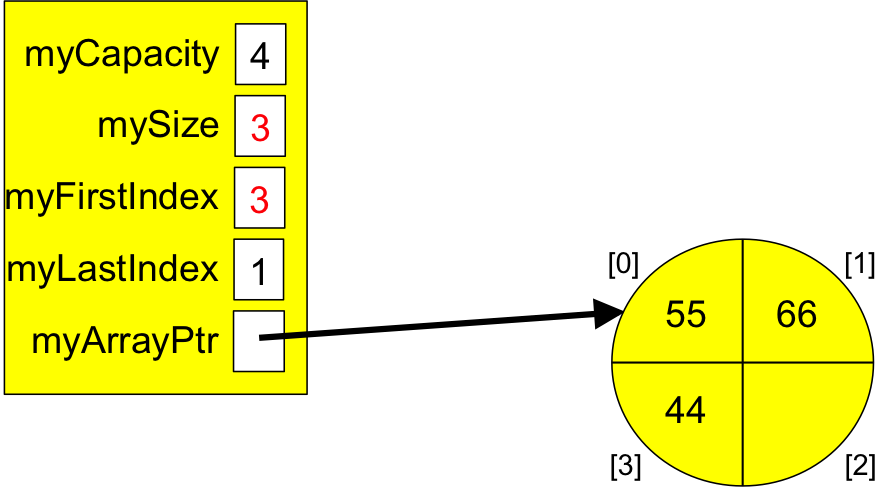Figure 15. Our Array-based Queue Containing 44, 55, and 66.

If we invoke remove() again, it should return the next-oldest item -- 44 -- and update its internal values as shown in Figure 16: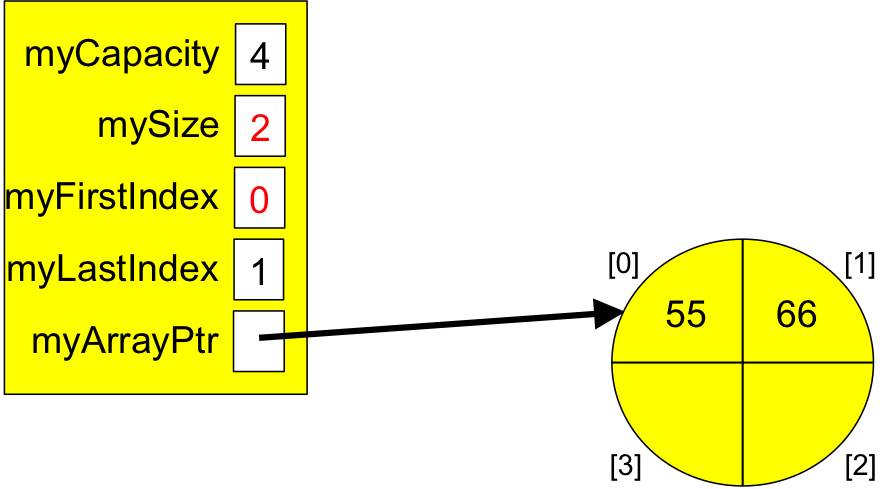Figure 16. Our Array-based Queue Containing 55, and 66.

Note that when remove() updates myFirstIndex, myFirstIndex must "wrap around" to myCapacity back to 0.

If we invoke remove() again, it should return the next-oldest item -- 55 -- and update its internal values as shown in Figure 17: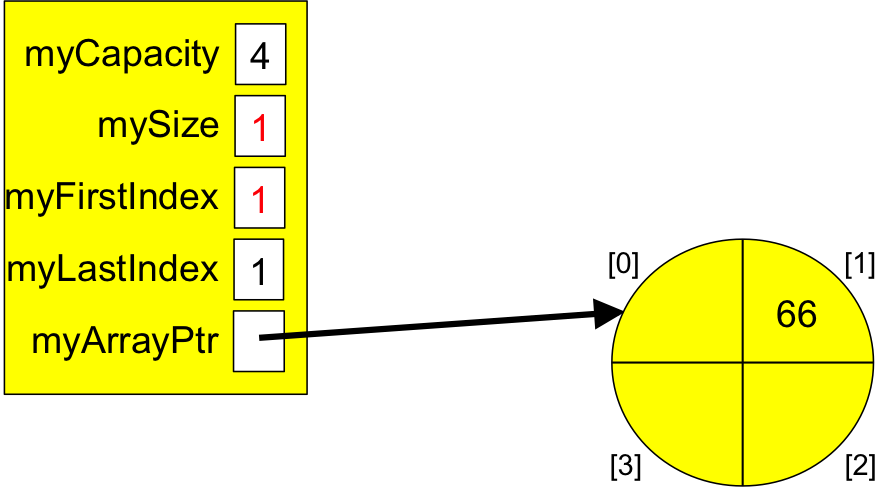Figure 17. Our Array-based Queue Containing 66.

If we invoke remove() once more, it should return the final item -- 66 -- leaving our queue as shown in Figure 18: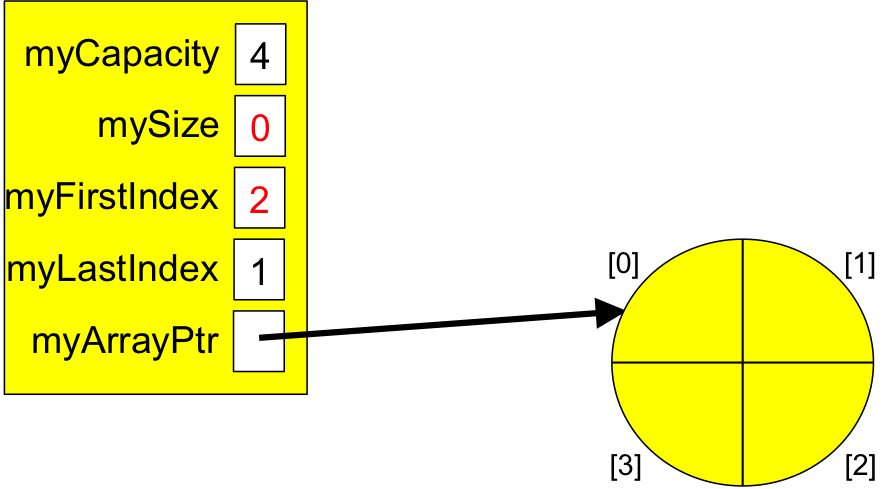Figure 18. Our Array-based Queue (Empty).

Some of the key things to observe are that the remove() operation:

• only changes myFirstIndex and mySize, not myLastIndex;
• needs to make the myFirstIndex member "wrap around" to 0 when it reaches myCapacity, which can be done using the modulus operator (%).

Using this information, go to ArrayQueue.cpp, and add a definition for remove() that will pass testRemove(). If you get stuck, here is a hint, but don't use it unless you have to.

Recompile and rerun your test. Continue when you pass the test.

Congratulations! You now have a working (dynamic-array-based) queue!

Close the ArrayQueue-related files, and open their LinkedQueue counterparts. Take a few minutes to look over the files, especially LinkedQueue.h. As you can see, our LinkedQueue class contains three instance variables:

```   unsigned mySize;       // number of items I contain
struct Node {
Item myItem;
Node * myNext;

Node(const Item& it, Node* next) { myItem = it; myNext = next; }
~Node()                          { delete myNext; }
};
Node * myFirstPtr;        // address of Node containing my oldest item
```
As before, we store the number of items in our queue in mySize. However, we store the address of a Node containing the oldest item (or NULL if our queue is empty) in myFirstPtr. Likewise, we store the address of the Node containing the newest item (or NULL if our queue is empty) in myLastPtr. This is sufficient, provided we use each Node's myNext member to chain together a sequence of nodes from *myFirstPtr to *myLastPtr, as shown in Figure 19: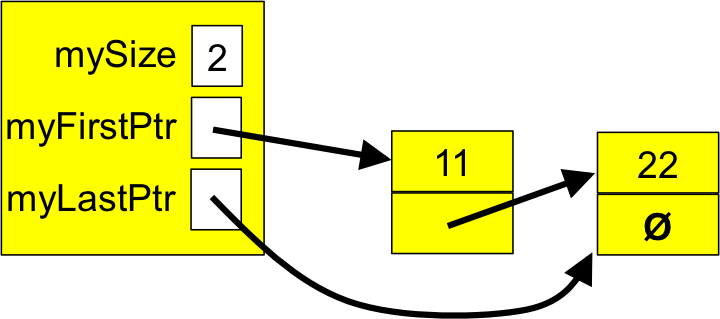Figure 19. Our Linked Queue (with two Items).

As with ArrayQueue, the provided LinkedQueue class already contain prototypes and definitions for:

• isEmpty() and isFull();
• getSize();
• a copy constructor;
• a destructor; and
• an assignment operator.
The copy constructor, destructor, and assignment operator are commented out. Leave them that way for now; we will uncomment them at the end.

Once again, run the project, and verify that everything works correctly at the outset.

In LinkedQueueTester.cpp, Uncomment the definition and call of LinkedQueueTester::testConstructor(). Take a few minutes to look over its definition. Study the tests it contains, specifically how it constructs a queue, and how this is different from our ArrayQueue. Our LinkedQueue constructor must initialize the members of a LinkedQueue with values appropriate for an initially empty queue.

Compile your project. You should see an error indicating that there is no constructor matching the calls in our test-method.

In LinkedQueue.h, add a prototype for this constructor to the class. Recompile and rerun. If you have done it correctly, you should now see a linking error, since our constructor has been prototyped but not defined.

With a bit of thought, it should be evident that defining our LinkedQueue constructor is a good bit simpler than our ArrayQueue constructor. Figure 20 shows how it should appear: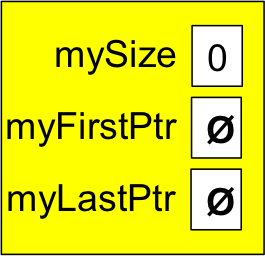Figure 20. Initializing a Linked Queue.

In LinkedQueue.cpp, add a definition for this constructor that will pass testConstructor(). If you get stuck, here is a hint, but don't use it unless you have to.

Recompile and rerun your test. Continue when your constructor passes the test.

The append(), getFirst(), and getLast() Methods

Before we can test the other operations, we need to be able to add items to a queue, so we now turn our attention to the append() method. Since adding items to a queue makes it non-empty, we will also define the getFirst() and getLast() methods.

In LinkedQueueTester.cpp, uncomment the call to and definition of the testAppend() method. Compile and run the test. You should see error messages indicating that append(), getFirst(), and getLast() do not exist.

In LinkedQueue.h, add a prototype for each of these methods to the class. Recompile. You should now get linking errors, since these methods have been declared but not defined.

The getFirst() Method. In LinkedQueue.cpp, add a definition for getFirst(). This definition should be fairly simple, throwing an EmptyQueueException if the queue is empty; otherwise, it should return the item in the Node whose address is stored in myFirst.

Save/compile, and verify that the linking error for getFirst() goes away. (The errors for getLast() and append() should still be there.)

The getLast() Method. Define getLast(). This method should follow the same pattern as getFirst(): if the queue is empty, throw an EmptyQueueException; otherwise, return the item of the Node whose address is stored in myLast.

Save/compile, and verify that the linking error for getLast() goes away. (The error for append() should still be there.)

The append() Method. Starting with an empty queue like that shown in Figure 20, suppose we append 11 to the queue. Our picture show change as shown in Figure 21: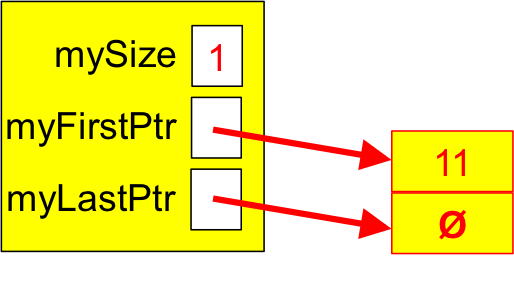Figure 21. A Linked Queue Containing One Item.

If we then append 22 to the queue, our picture changes as shown in Figure 22: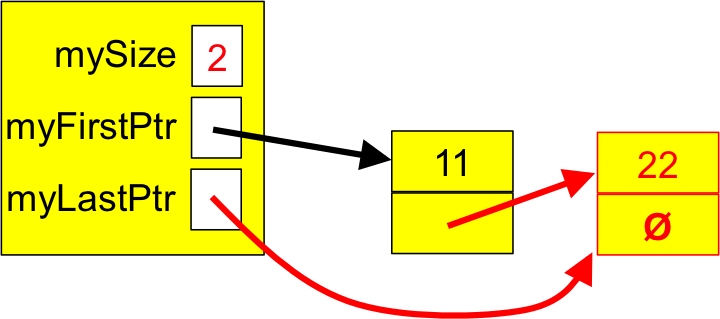Figure 22. A Linked Queue Containing Two Items.

If we then append 33 to the queue, our picture changes as shown in Figure 23: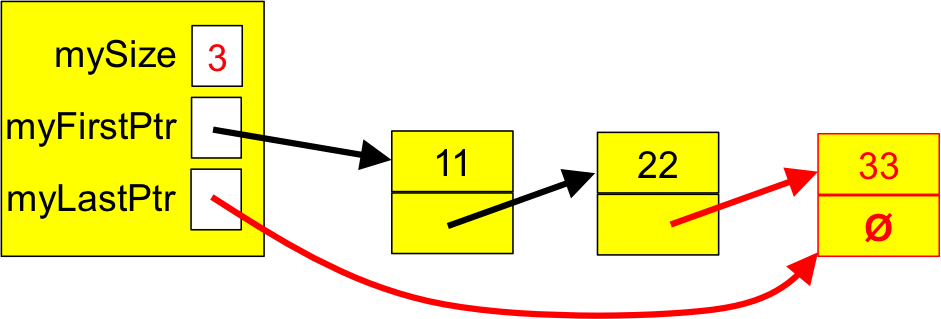Figure 23. A Linked Queue Containing Three Items.

Note that conceptually, appending to a linked queue is somewhat simpler than appending to an array-based queue, because a linked queue has no fixed capacity -- the only time it will ever be full is if our program runs out of memory! If this happens, our attempt to allocate the new node will throw a bad_alloc() exception, so we can catch that exception and use it to throw a FullQueueException.

Using this information, define the append() method. Save/recompile, and verify that what you have written compiles without errors.

Run the tests. If you pass the tests, congratulations! If not, go back and fix your append(), getFirst() and/or getLast() methods. If you get stuck, here is a hint for append(), but only use it if you have to.

Continue when your methods pass all the tests.

The remove() Method

Now that we are able to add items to our LinkedQueue, and view the first and last items in the queue, all that remains is to build the remove() operation.

In LinkedQueueTester.cpp, uncomment the call to and definition of the testRemove() method. Compile and run the test. You should see an error message indicating that remove() does not exist.

In LinkedQueue.h, add a prototype for remove() to the class. Recompile and rerun. You should now see a linking error, since remove() has been prototyped but not defined.

To implement remove():

1. It's clear that we cannot remove items from an empty queue (see Figure 20), so we might begin by noting that we need to throw an EmptyQueueException if the queue is empty.
2. For cases where the queue is not empty, let's start with our scenario from Figure 23. Here it is again:Figure 23. A Linked Queue Containing Three Items.

Removing an item from this queue should retrieve the oldest item (11), dispose of that node, and return the 11, leaving our queue as shown in Figure 24:Figure 24. A Linked Queue Containing Two Items.

Likewise, removing an item from this queue should retrieve the oldest item (22), dispose of that node, and return the 22, leaving our queue as shown in Figure 25: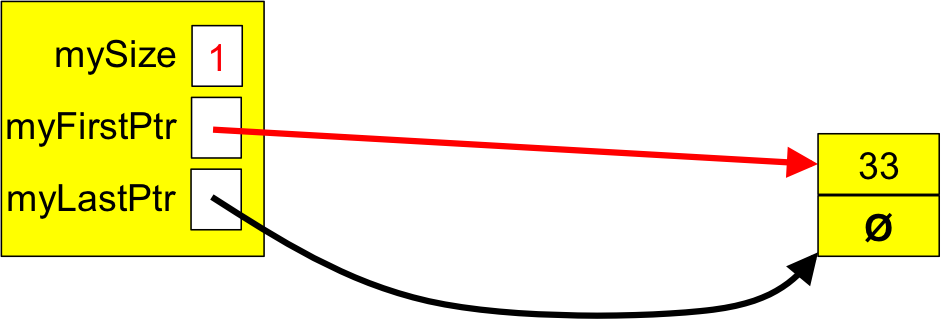Figure 25. A Linked Queue Containing One Item.

Finally, removing an item from this queue should retrieve the final item (33), dispose of that node, and return the 33, leaving our queue as shown in Figure 26: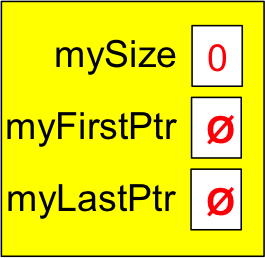Figure 26. A Linked Queue Containing No Items.

Using this information, go to LinkedQueue.cpp, and add a definition for remove() that will pass testRemove(). If you get stuck, here is a hint, but don't use it unless you have to.

Recompile and rerun your test. Continue when you pass the test.

Congratulations! You now have a working (linked-list-based) queue!

The Other Operations

Now that the primary queue operations have passed their tests, uncomment the remaining test-methods and verify that the other ArrayQueue and LinkedQueue operations pass their tests.

Congratulations! You now have full-featured ArrayQueue and LinkedQueue classes at your disposal! Each requires just O(1) to append() or remove() an item. That said, the ArrayQueue operations are faster than their LinkedQueue counterparts because:

• Each call to LinkedQueue::append() allocates memory,
• Each call to LindexQueue::remove() deallocates memory,
and allocating/deallocating memory takes time.

A LinkedQueue may also use more memory/space than an ArrayQueue to store a given number of items, so it can be more space-expensive.

However, a LinkedQueue never gets "full" unless you run out of dynamic memory. This makes it much more convenient to use, since you never have to worry about resizing it.